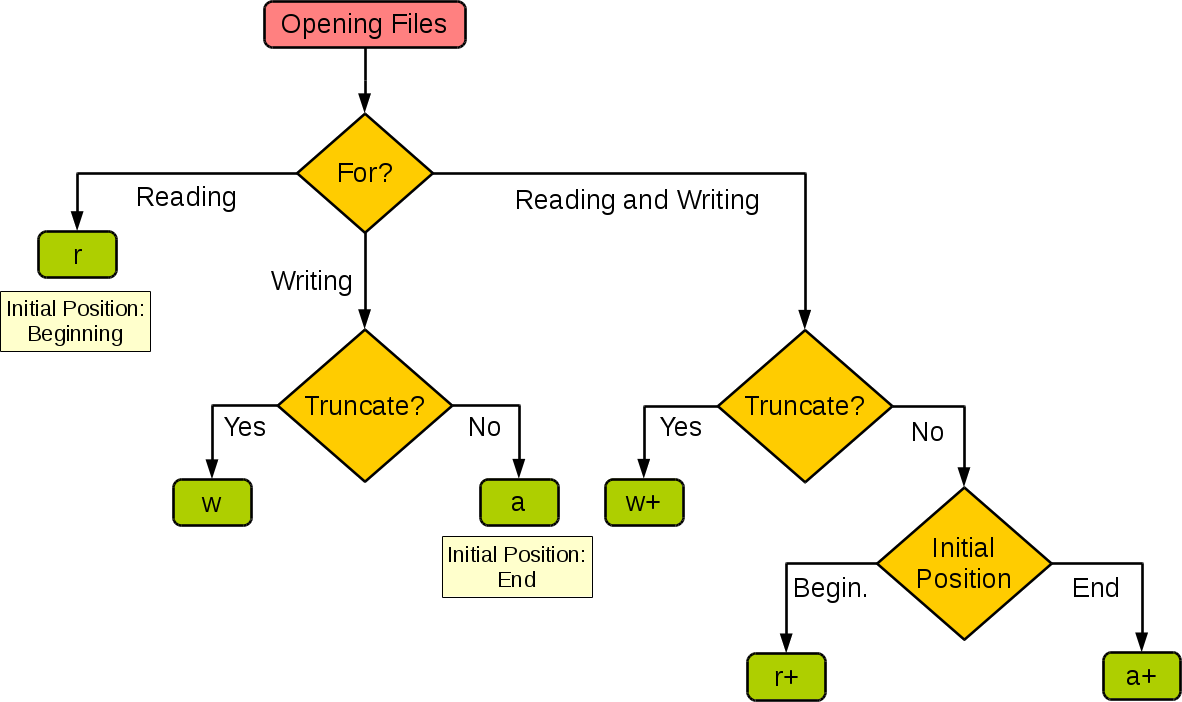## Python基操

• 对Python常用的基础知识整理回顾
• 大部分操作使用代码块说明 ，IDE使用PyCharm

### 字符串

• Python3中统一采用Unicode编码 ，也封装了字符串类`str`
``````mystr = 'RoyKun'
result = mystr.index('R')   # 获取字符索引，没找到会崩溃
print(result)	# 0

result = mystr.find('K')   # 获取字符索引，如果没找到返回-1
print(result)	# 3	区分大小写哦！

result = mystr.count('u')   # 字符出现的个数
print(result)	# 1

result = len(mystr)     # 返回字符串长度
print(result)	# 6

result = mystr.replace('u','n')     # 字符替换
print(result)	# RoyKnn
print(mystr)	# RoyKun

mystr = 'apple,pear,orange'
result = mystr.split(',')    # 分割数据
print(result)   # ['apple', 'pear', 'orange']	得到列表

mystr = 'aabccb'
result = mystr.partition('b')# 以指定字符串分割为3部分
print(result)   # ('aa', 'b', 'ccb')	得到元祖	，只会以第一个 , 分割

myurl = 'http://www.baidu.com'
result = myurl.startswith('http')   # 是否以指定数据开头
print(result)   # True

myurl = 'http://www.baidu.com'
result = myurl.endswith('xxx')   # 是否以指定数据开头
print(result)	# False

mystr = 'k'
result = mystr.join('Roy')  # 以指定字符串拼接数据
print(result)   # Rkoky
mystr = mystr +'Roy'
print(mystr )	# kRoy

mystr = ' Roy '
result = mystr.strip(' ')   # Roy	去除两边指定字符
# result = mystr.lstrip(' ')
# result = mystr.rstrip(' ')
print(result)
``````
• 注意有些是引用，直接操作；有些会返回新的对象

### 常见数据类型及操作

• 列表

``````# 列表：以中括号表现形式的数据集合，可以放任意类型的数据
# 因此	，列表本质是一个指针数组
my_list = [1, 'app', True]
print(type(my_list))    # <class 'list'>
print(my_list[-2])      # app

my_list.append('Roy')   # 追加数据
my_list.insert(1, 'kun')    # 指定位置插入数据	[1, 'kun', 'app', True, 'Roy']

mylist2 = ['watermelon', '草莓']
my_list.extend(mylist2)     # 添加列表元素（扩展）
print(my_list)	# [1, 'app', True, 'watermelon', '草莓']

my_list.remove('草莓')    # 删除指定元素
del my_list      # 根据下标删除元素	['app', True, 'watermelon']

result = my_list.pop(1)  # 删除数据并返回数据值，如果不传参 默认删除 -1 位置
# 即 list默认是个栈，后进先出
print(result)

# 列表生成式
my_list = [value for value in range(1,6)]
print(my_list)  # [1, 2, 3, 4, 5] 包头不包尾
my_list = [value+"Hello" for value in ['ab','abc']]
print(my_list)
my_list = [(x,y) for x in range(1,3) for y in range(2,4)]	# range包头不包尾
print(my_list)  # [(1, 2), (1, 3), (2, 2), (2, 3)] 等价于两层for循环
``````
• 元祖

``````# 元祖：以小括号形式表现的数据集合	，可以存任意类型的数据
# 不能对元祖进行数据修改
my_tuple = (1, 2, 'Roy',[3,4])
print(my_tuple)
# my_tuple = 2   # 不能直接修改
# TypeError: 'tuple' object does not support item assignment
my_tuple.append(5)
print(my_tuple)	# (1, 2, 'Roy', [3, 4, 5])
# 如果存储的元素本身是可变的，那可以改变这个元素

# 如果只有一个元素需要添加逗号，不然会判断为其他类型
my_tuple2 = (1,)
print(type(my_tuple2)) # <class 'tuple'>
my_tuple2 = (1)		   # <class 'int'>
``````
• 字典

``````# 字典：以大括号形式表现的数据集合	，元素使用键值对表示
# 字典中的数据是无序的（输出顺序不定）
my_dict = {'name':'Roy', 'age':18, 'hobby':'fuck'}
print(my_dict)
result = my_dict.pop('age') # 删除元素，必须指定key

# 使用dict函数
dict2 = dict(a='a', b='b', t='t')	# 一般传入的是可迭代对象
print(dict2)
dict(zip(['one', 'two', 'three'], [1, 2, 3]))
dict([('one', 1), ('two', 2), ('three', 3)])
``````
• 集合

``````# 集合set：以大括号表示的数据集合，无序	，且不能重复
my_set = {1, 2, 'Roy'}
print(type(my_set))     # <class 'set'>

my_set.remove(1)    # 删除指定元素，元素必须存在否则报错
print(my_set)
print(my_set)

my_set2 = set()     # 若没有元素时
print(type(my_set2))	# <class 'set'>

print(my_set2)
# 无序，也就是说不能根据下标进行操作
``````
• 字典和集合都用大括号=={ }==

• 列表 、元祖 、集合都叫做数据容器，可以相互转换

``````my_list = [1,2,2]
my_tuple = (4,5)
my_set = {'name', 'age'}
# 列表转集合
result = set(my_list)   # 会对元素去重
# 元祖转集合
result = set(my_tuple)
# 列表转元祖
result = tuple(my_list)
# 集合转元祖
result = tuple(my_set)
# 集合转列表
result = list(my_set)
# 元祖转列表
result = list(my_tuple)
``````
• `enumerate()`函数用于将一个可遍历的数据对象(如元组、列表、集合或字符串)组合为一个索引序列

• 也是后面拆包的常用操作

``````for index, value in enumerate(['apple', 'banana']):
print(index,value)		# 在命令行执行要加空格
# 0 apple		加序号
# 1 banana
``````
• 针对字典可以使用 `item()` 方法迭代

``````# 针对字典
my_dict = {'name':'Roy', 'age':18, 'hobby':'fuck'}
for key, value in my_dict.items():	# 这个就是key了	，而不是序号！
print(key,value)
for keys in my_dict.keys():  # 当然，还有keys()方法
print(keys)
``````
• 字典 、列表、字符串是最常见的数据结构，且都是可迭代对象 ，后面会介绍迭代器

### 函数相关操作

• 函数传参

``````# 不定长参数函数
def fun(a, b, *args, **kwargs):# a,b是必传参数
print(a)
print(b)
print(args)
print(kwargs)

fun(1, 2, 3, 4, name = "hello", age = 20)
# 1
# 2
# (3, 4)    元祖封装  为什么不用列表呢？
# {'name': 'hello', 'age': 20}  字典封装

fun(1, 2, 3, 4, name = "hello", age = 20, 18) # SyntaxError: positional argument follows keyword argument  语法错误，参数位置不正确（这让人家没法归类啊！）
``````
• 递归函数

``````# 递归函数：传递回归，即在函数内部再次调用函数
def cal_num(num):   # 计算阶乘
if  not isinstance(num, int):
return 'error input'
if num == 1:    # 结束递归的条件（递归出口）
return 1
else:
return num * cal_num(num-1)

cal_num(5)

import sys
result = sys.getrecursionlimit()    # 获取最大递归次数
print(result)   # 1000
sys.setrecursionlimit(1100)     # 设置最大递归次数
``````
• 程序本质上就是：循环、判断 、递归

• 匿名函数

``````# 匿名函数：没有名称的函数
# 由于这个函数只在这里用一下	，所以没有必要单独定义，可以简化代码
# func接收函数即可，需要传递函数作为参数时	，直接写lambda表达式即可
func = lambda x,y: x+y      # 前面是参数；后面只能写一句表达式，返回计算结果
result = func(2,4)
print(result)

func1 = lambda num: True if num%2 == 0 else False	# 判断奇偶 T要大写，放在前面！
print(func1(5))

list2 = [{'name':'roy','age':22},{'name':'kun','age':20}]   # 对字典列表进行排序
list2.sort(key=lambda item: item['age'])	# key= reverse= 是关键字
# 默认False	，升序！
print(list2)	# item代表列表中的每个字典，sort函数内部循环获取所有age值排序
``````
• 语法就记一下吧：简单运算、bool判断、排序（传递关键字）

• 高阶函数

``````# 高阶函数
# 参数是函数或者返回函数的函数
def test(new_func):
new_func()  # 调用
def inner():
print('内部函数')
return inner
def show():
print('我是个函数，但我在这做函数参数')
func = test(show)
``````

### 装饰器

• 闭包：是高级函数

``````# 函数嵌套时，内部函数使用外部函数的参数或变量	，最终返回这个内部函数，叫做闭包
def exfunc(msg,num):
def refunc():
print(msg*num)
return refunc   # 注意不带括号

# 闭包的好处是可以根据参数得到新函数，从而适应不同需求
func = exfunc('roy',2)
func()		# royroy
print(func) # <function exfunc.<locals>.refunc at 0x000002572A4AFE58>
``````
• 装饰器：函数引用指向原函数 ，原函数名接收闭包，实现功能扩展

``````# 装饰器本质上是一个函数，可以对原函数的功能进行扩展	，不影响原函数的定义和调用
# 装饰器的实现要用到闭包

# 通用装饰器：可以修饰带参数的和不带参数的函数，有返回值的和无返回值的函数
def decorator(func):    # 最外层，传递函数名
def inner(*args, **kwargs):	# 内层	，接收原函数参数
print('这是对被装饰函数扩展的操作')	# 扩展部分
return func(*args, **kwargs)    # 需要设置原函数的参数，对于有返回值的函数需要return；总之，加上是没错的！
return inner
# 上面是两层装饰器	，如果装饰器也带参数呢？就需要三层

@decorator  # 语法糖，相当于执行 sum = decorator(sum) 接收闭包，扩展了原函数
def sum(num1,num2):
print(num1 + num2)
sum(1,3)	# 这是对被装饰函数扩展的操作

@decorator
def test():
print('Roy')
test()  # 这里函数不带有参数
``````
• 切片（取元素）

``````# 针对字符串，类似numpy的矩阵操作
str = 'RoyKun'
result = str[0:5:1] # 起始下标：结束下标：步长 （不包含结束下标）
print(result)   # RoyKu

result = str[-1:-4:-1]  # nuK
print(result)
``````

### 文件操作

• 主要用到`open`函数和`os`

``````# 读取键盘输入	，标准输入读入一行文本
str = input("请输入：")
print "你输入的内容是: ", str
``````
• 这里均是在Linux环境中的操作

``````# 打开文件使用open函数
fileObject = open('1.txt', 'r', encoding='UTF-8')# 在Windows上使用utf-8编码格式
# 使用open函数相当于创建了一个file对象，包含常用方法：
string = 'RoyKun'
fileObject.write(string)	# 写入字符串
string = fileObject.read(6)	# 读取文件内容 参数count是要从已打开文件中读取的字节计数
position = fileObject.tell() # 查找当前位置
fileObject.close()	# 关闭对象
# 在python3中默认支持中文编码(都是Unicode)，也可以在代码首行使用：-*- coding: UTF-8 -*-	指定编码格式
# 使用'b'模式时	，不需要指定编码格式，同时读取数据需要使用decode()解码
``````
• 其他方法

``````# 刷新文件内部缓冲，直接把内部缓冲区的数据立刻写入文件, 而不是被动的等待输出缓冲区写入
file.flush()

# 读取整行	，包括 "\n" 字符

# 读取所有行并返回列表，若给定sizeint>0，则是设置一次读多少字节	，这是为了减轻读取压力

# 截取文件，截取的字节通过size指定，默认为当前文件位置
file.truncate([size])

# 移动文件读取指针到指定位置
file.seek(offset[, whence])
``````
• 对于打开文件的模式总结如下图：• 文件对象还包含以下属性：

属性 描述
file.closed 返回true如果文件已被关闭 ，否则返回false 。
file.mode 返回被打开文件的访问模式 。
file.name 返回文件的名称。
file.softspace 如果用print输出后 ，必须跟一个空格符，则返回false。否则返回true 。
• os模块提供了帮你执行文件处理操作的方法，比如重命名和删除文件

``````import os

# 列出当前目录下的文件
os.listdir(os.getcwd())

# 重命名文件test1.txt到test2.txt。
os.rename( "test1.txt", "test2.txt" )

# 删除文件
os.remove(file_name)

# 创建一个新目录
os.mkdir("newdir")

# 将当前目录改为"/home/newdir"
os.chdir("/home/newdir")

# 显示当前的工作目录
os.getcwd()

# 删除目录	，它的所有内容应该先被清除
os.rmdir('dirname')
``````
• 小结：`open` 函数和 `os` 模块最常用，很多使用也用到os定位路径

### json序列化

• JSON (JavaScript Object Notation) 是一种轻量级的数据交换格式，它基于ECMAScript的一个子集

• 使用 `json` 模块对数据进行编解码 ，主要包括

• `json.dumps()` 转换为json数据（字符串 ）
• `json.loads()` json数据转换成python类型数据
• Python 编码为 JSON 后类型都为string：

``````import json

data = [ { 'a' : 1, 'b' : 2, 'c' : 3, 'd' : 4, 'e' : 5 } ]

data_json = json.dumps(data)
print(type(data_json))  # <class 'str'>